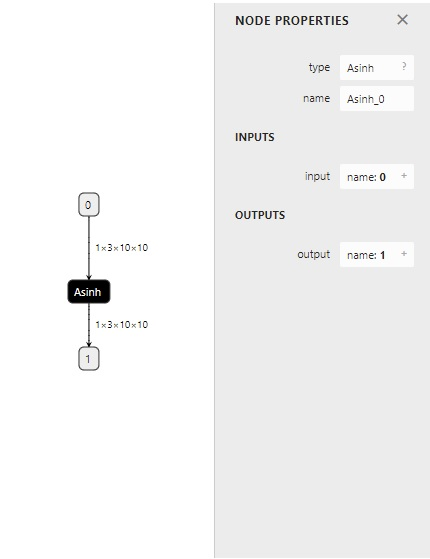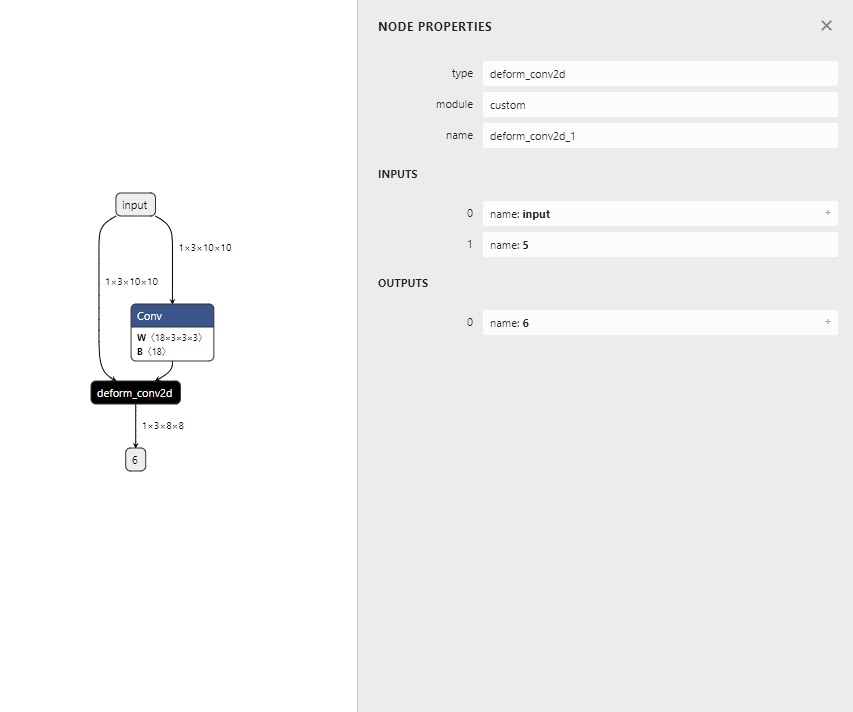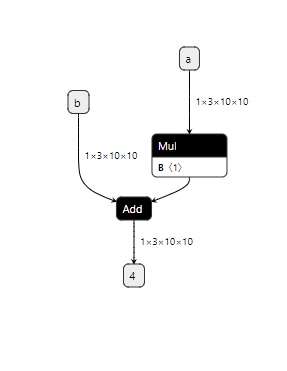Shortcuts

# 第四章：在 PyTorch 中支持更多 ONNX 算子¶

• 算子在 PyTorch 中有实现

• 有把该 PyTorch 算子映射成一个或多个 ONNX 算子的方法

• ONNX 有相应的算子

• PyTorch 算子

• 组合现有算子

• 添加 TorchScript 算子

• 添加普通 C++ 拓展算子

• 映射方法

• 为 ATen 算子添加符号函数

• 为 TorchScript 算子添加符号函数

• ONNX 算子

• 使用现有 ONNX 算子

• 定义新 ONNX 算子

## 支持 ATen 算子¶

ATen 是 PyTorch 内置的 C++ 张量计算库，PyTorch 算子在底层绝大多数计算都是用 ATen 实现的。

### 获取 ATen 中算子接口定义¶

def asinh(input: Tensor, *, out: Optional[Tensor]=None) -> Tensor: ...


### 添加符号函数¶

def symbolic(g: torch._C.Graph, input_0: torch._C.Value, input_1: torch._C.Value, ...):


g 有一个方法 op。在把 PyTorch 算子转换成 ONNX 算子时，需要在符号函数中调用此方法来为最终的计算图添加一个 ONNX 算子。其定义如下：

def op(name: str, input_0: torch._C.Value, input_1: torch._C.Value, ...)


from torch.onnx.symbolic_registry import register_op

def asinh_symbolic(g, input, *, out=None):
return g.op("Asinh", input)

register_op('asinh', asinh_symbolic, '', 9)


def asinh(input: Tensor, *, out: Optional[Tensor]=None) -> Tensor: ...


register_op('asinh', asinh_symbolic, '', 9)


register_op的第一个参数是目标 ATen 算子名，第二个是要注册的符号函数，这两个参数很好理解。第三个参数是算子的“域”，对于普通 ONNX 算子，直接填空字符串即可。第四个参数表示向哪个算子集版本注册。我们遵照 ONNX 标准，向第 9 号算子集注册。值得注意的是，这里向第 9 号算子集注册，不代表较新的算子集（第 10 号、第 11 号……）都得到了注册。在示例中，我们先只向第 9 号算子集注册。

import torch

class Model(torch.nn.Module):
def __init__(self):
super().__init__()

def forward(self, x):

from torch.onnx.symbolic_registry import register_op

def asinh_symbolic(g, input, *, out=None):
return g.op("Asinh", input)

register_op('asinh', asinh_symbolic, '', 9)

model = Model()
input = torch.rand(1, 3, 10, 10)
torch.onnx.export(model, input, 'asinh.onnx')### 测试算子¶

import onnxruntime
import torch
import numpy as np

class Model(torch.nn.Module):
def __init__(self):
super().__init__()

def forward(self, x):

model = Model()
input = torch.rand(1, 3, 10, 10)
torch_output = model(input).detach().numpy()

sess = onnxruntime.InferenceSession('asinh.onnx')
ort_output = sess.run(None, {'0': input.numpy()})

assert np.allclose(torch_output, ort_output)


## 支持 TorchScript 算子¶

1. 获取原算子的前向推理接口。

2. 获取目标 ONNX 算子的定义。

3. 编写符号函数并绑定。

### 使用 TorchScript 算子¶

import torch
import torchvision

class Model(torch.nn.Module):
def __init__(self):
super().__init__()
self.conv1 = torch.nn.Conv2d(3, 18, 3)
self.conv2 = torchvision.ops.DeformConv2d(3, 3, 3)

def forward(self, x):
return self.conv2(x, self.conv1(x))


m.def(TORCH_SELECTIVE_SCHEMA(
"torchvision::deform_conv2d(Tensor input,
Tensor weight,
Tensor offset,
......


### 自定义 ONNX 算子¶

g.op("custom::deform_conv2d, ...)


PyTorch 在运行 g.op() 时会对官方的算子做检查，如果算子名有误，或者算子的输入类型不正确， g.op() 就会报错。为了让我们随心所欲地定义新 ONNX 算子，我们必须设定一个命名空间，给算子取个名，再定义自己的算子。

@parse_args("v", "v", "v", "v", "v", "i", "i", "i", "i", "i", "i", "i", "i", "none")
def symbolic(g,
input,
weight,
offset,
bias,
stride_h, stride_w,
dil_h, dil_w,
n_weight_grps,
n_offset_grps,
return g.op("custom::deform_conv2d", input, offset)


register_custom_op_symbolic("torchvision::deform_conv2d", symbolic, 9)


import torch
import torchvision

class Model(torch.nn.Module):
def __init__(self):
super().__init__()
self.conv1 = torch.nn.Conv2d(3, 18, 3)
self.conv2 = torchvision.ops.DeformConv2d(3, 3, 3)

def forward(self, x):
return self.conv2(x, self.conv1(x))

from torch.onnx import register_custom_op_symbolic
from torch.onnx.symbolic_helper import parse_args

@parse_args("v", "v", "v", "v", "v", "i", "i", "i", "i", "i", "i", "i", "i", "none")
def symbolic(g,
input,
weight,
offset,
bias,
stride_h, stride_w,
dil_h, dil_w,
n_weight_grps,
n_offset_grps,
return g.op("custom::deform_conv2d", input, offset)

register_custom_op_symbolic("torchvision::deform_conv2d", symbolic, 9)

model = Model()
input = torch.rand(1, 3, 10, 10)
torch.onnx.export(model, input, 'dcn.onnx')### 为 PyTorch 添加 C++ 拓展¶

// my_add.cpp

#include <torch/torch.h>

{
return 2 * a + b;
}

PYBIND11_MODULE(my_lib, m)
{
}


from setuptools import setup
from torch.utils import cpp_extension

cmdclass={'build_ext': cpp_extension.BuildExtension})


python setup.py develop


### 用 torch.autograd.Function 封装¶

import torch
import my_lib

@staticmethod
def forward(ctx, a, b):

@staticmethod
def symbolic(g, a, b):
two = g.op("Constant", value_t=torch.tensor())
a = g.op('Mul', a, two)


PyTorch 会自动调度该函数，合适地执行前向和反向计算。对模型部署来说，Function 类有一个很好的性质：如果它定义了 symbolic 静态方法，该 Function 在执行 torch.onnx.export() 时就可以根据 symbolic 中定义的规则转换成 ONNX 算子。这个 symbolic 就是前面提到的符号函数，只是它的名称必须是 symbolic 而已。

forward 函数中，我们用 my_lib.my_add(a, b) 就可以调用之前写的C++函数了。这里 my_lib 是库名，my_add 是函数名，这两个名字是在前面C++的 PYBIND11_MODULE 中定义的。

symbolic 函数中，我们用 g.op() 定义了三个算子：常量、乘法、加法。这里乘法和加法的用法和前面提到的 asinh 一样，只需要根据 ONNX 算子定义规则把输入参数填入即可。而在定义常量算子时，我们要把 PyTorch 张量的值传入 value_t 参数中。

my_add = MyAddFunction.apply

def __init__(self):
super().__init__()

def forward(self, a, b):


### 测试算子¶

model = MyAdd()
input = torch.rand(1, 3, 10, 10)
torch_output = model(input, input).detach().numpy()

import onnxruntime
import numpy as np
ort_output = sess.run(None, {'a': input.numpy(), 'b': input.numpy()})

assert np.allclose(torch_output, ort_output)import torch
import my_lib

@staticmethod
def forward(ctx, a, b):

@staticmethod
def symbolic(g, a, b):
two = g.op("Constant", value_t=torch.tensor())
a = g.op('Mul', a, two)

def __init__(self):
super().__init__()

def forward(self, a, b):

input = torch.rand(1, 3, 10, 10)
torch_output = model(input, input).detach().numpy()

import onnxruntime
import numpy as np
ort_output = sess.run(None, {'a': input.numpy(), 'b': input.numpy()})

assert np.allclose(torch_output, ort_output)


## 总结¶

• ATen 是 PyTorch 的 C++ 张量运算库。通过查询 torch/_C/_VariableFunctions.pyi 和 torch/nn/functional.pyi，我们可以知道 ATen 算子的 Python 接口定义。

• 用 register_op 可以为 ATen 算子补充注册符号函数

• 用 register_custom_op_symbolic 可以为 TorchScript 算子补充注册符号函数

• 如何在 PyTorch 里添加 C++ 拓展

• 如何用 torch.autograd.Function 封装一个自定义 PyTorch 算子

• 如何编写符号函数 symbolic(g, …)。

• 如何用 g.op() 把一个 PyTorch 算子映射成一个或多个 ONNX 算子，或者是自定义的 ONNX 算子。

## 上期习题解答¶

1. PyTorch 目前没有支持 ONNX 的 Asinh 算子。我们在 torch.onnx.symbolic_opset9.py 中搜索不到 Asinh 的相关内容。

2. 通过在 torch.onnx.symbolic_opset11.py 搜索 BitShift，我们可以发现 PyTorch 在 __lshift___rshift_ 里用到了ONNX的 BitShift 算子。当输入类型为 Byte 时，PyTorch会把算子直接翻译翻译 BitShift，以代替乘除 2 的次幂的操作。

3. 对应 Resize 算子的第3个参数（g.op() 的第4个参数）scales。原来的 scales 传入 g.op() 前会经过 _interpolate_get_scales_if_available() 函数，一定会被转换成一个常量。为了让 scales 由输入决定，我们直接把输入参数中的 scales 传入 g.op()

© Copyright 2021-2024, OpenMMLab. Revision 660af62b.

Built with Sphinx using a theme provided by Read the Docs.
Versions
latest
stable
v1.3.0
v1.2.0
v1.1.0
v1.0.0
0.x
v0.14.0